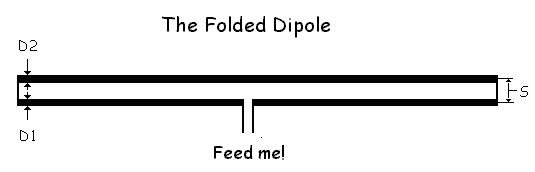D1 and D2 are the diameters of the conductors. S is the distance between the centers of the conductors.

### Output Values

D2/D1 =
S/D2 =
R =
Assuming that the characteristic impedance of a dipole is as defined in the Input Values section, the input impedance of this folded dipole is:
Ohms.

### Input Values

Change the value, move the pointer out of the field,
and click to re-calculate.
Or just hit the RECALC button.
D1 =
D2 =
S =
Assumed Dipole Impedance = Ohms